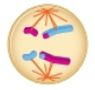Chapter 12, Problem 7SQ

Chapter
Section

Textbook ProblemThe cell in the diagram to the right is in anaphase I, not anaphase II. I know this because __________.Summary Introduction

Introduction: Meiotic division is a division that occurs in a cell that typically lessens the number of chromosomes present in the parent cell by half. Meiotic divisions are more complex than the mitosis. Here, the cell divides twice the times. The first division of diploid cell gives two diploid daughter cells (Meiosis I) and the next division reduces the chromosome number by half that later on give rise to four haploid cells. Every round of division contains four stages that include:  the initial prophase metaphase, anaphase and the final telophase.

Still sussing out bartleby?

Check out a sample textbook solution.

See a sample solution